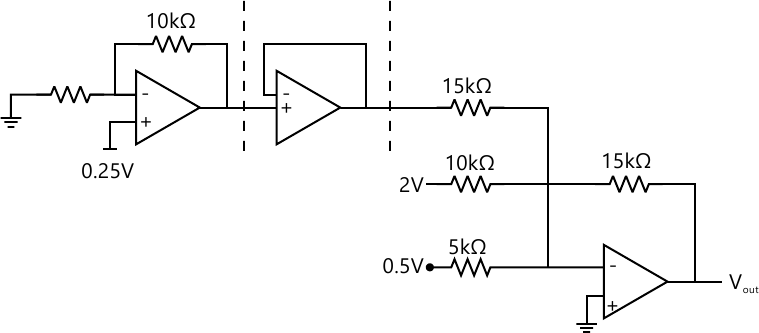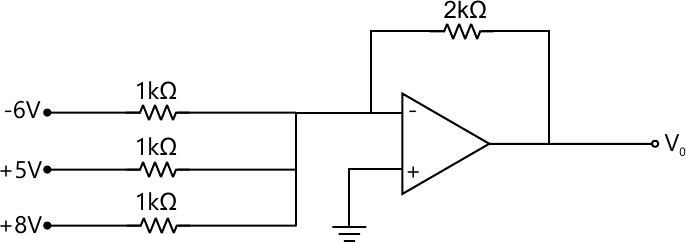MORE IN Basic Electronics
VTU First Year Engineering (C Cycle) (Semester 1)
Basic Electronics
December 2007
Total marks: --
Total time: --
INSTRUCTIONS
(1) Assume appropriate data and state your reasons
(2) Marks are given to the right of every question
(3) Draw neat diagrams wherever necessary

1(a) With appropriate circuit diagram explain the DC load line analysis of semi conductor diode.
5 M
1(b) In a full wave rectifier, the input is from 30-0-30 V transformer. The load and diode forward resistances are 100? and 10? respectively. Calculate the average voltage, dc output power, ac input power, rectification efficiency and percentage regulation.
5 M
1(c) Explain the working of positive clamping circuit.
5 M
1(d) In a Common Emitter transistor circuit if ?= 100 and IB= 50?A,compute the values of ?, IEand Ic.
5 M

2(a) With a neat circuit diagram and waveforms explain t he working of full wave bridge rectifier and show that its ripple factor is 0.48.
8 M
2(b) Draw the common emitter circuit and sketch the input and output characteristics. Also explain active region, cutoff region and saturation region by indicating them on the characteristic curve.
7 M
2(c) Design Zener voltage regulator for the following sp ecifications:Input Voltage=10V±20%, Output Voltage=5V,IL=20mA,Izmin=5mA and Izmax=80mA.
5 M

3(a) With a neat circuit diagram explain the Voltage Divider Bias circuit by giving its exact analysis.
8 M
3(b) Explain the characteristics of an Ideal Op-Amp. Mention some of the applications of Op-Amp.
6 M
3(c) Determine the operating point for a silicon transisto r biased by base bias method with ?= 100, RB= 500K?RC= 2.5K? and VCC= 20V. Also draw the DC load line.
6 M

4(a) Explain how Op-Amp can be used as
i)Integrator ii) Inverting Summer and iii) Voltage Follower
10 M
4(b) Find the output of the following Op-Amp Circuits.

i)ii)10 M

5(a) State and prove DeMorgan's Theorems for three variables.
4 M
5(b) Realize two input Ex-OR gate using only NAND gates.
5 M
6 M
5(d) With the help of switching circuit, Input/output waveforms and truth table explain the operation of a NOT Gate.
5 M

6(a) Design a logic circuit using basic gates with three inputs A,B, C and output Y that goes low only when A is high and B and C are different
5 M
5 M
6(c) (i)Subtract (1111.101) 2from (1001.101)2 using 1's compliment method.
ii)Subtract (11101.111)2from (11111.101)2using 2's compliment method.
5 M
6(d) Simplify Y=AB+ AC+ABC(AB+ C)
5 M

7(a) Define Flip Flop. Give the difference between Latch and Flip Flop.
4 M
7(b) Explain the working of LVDT.
6 M
7(c) With the help of logic diagram and truth table explain the working of clocked RS Flip Flop.
5 M
7(d) List the differences between Microprocessor and Microcontroller.
5 M

8(a) With a neat block diagram explain the architecture of 8085 Microprocessor
9 M
8(b) What is a Transducer? Distinguish between active and passive transducers.
5 M
8(c) Explain i) Hall Effect ii) Seebeck Effect iii) Peltier Effect
6 M

9(a) What are the commonly used frequency ranges in communication systems? Mention the applications of each range.
4 M
9(b) Define AM. Draw the AM signal and its Spectrum. Derive an expression for total power in an AM signal.
6 M
9(c) Calculate the percentage power saving when one side band and carrier is suppressed in an AM signal with modulation index equal to 1.
5 M
9(d) With a network diagram explain the working of typical switched telephone system.
5 M

10(a) With a block diagram explain typical cellular mobile radio unit.
5 M
10(b) What is ISDN? Explain the services of ISDN.
5 M
10(c) Give the comparison between AM and FM.
4 M
10(d) With a neat block diagram explain optical fibre communication system.
6 M

More question papers from Basic Electronics